# Multiplication and Division

These activities are part of our Primary collections, which are problems grouped by topic.### Multiply Multiples 2

##### Age 7 to 11Challenge Level

Can you work out some different ways to balance this equation?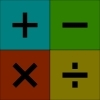### Highest and Lowest

##### Age 7 to 11Challenge Level

Put operations signs between the numbers 3 4 5 6 to make the highest possible number and lowest possible number.### Which Is Quicker?

##### Age 7 to 11Challenge Level

Which is quicker, counting up to 30 in ones or counting up to 300 in tens? Why?### What Do You Need?

##### Age 7 to 11Challenge Level

Four of these clues are needed to find the chosen number on this grid and four are true but do nothing to help in finding the number. Can you sort out the clues and find the number?### Multiply Multiples 3

##### Age 7 to 11Challenge Level

Have a go at balancing this equation. Can you find different ways of doing it?### Zios and Zepts

##### Age 7 to 11Challenge Level

On the planet Vuv there are two sorts of creatures. The Zios have 3 legs and the Zepts have 7 legs. The great planetary explorer Nico counted 52 legs. How many Zios and how many Zepts were there?### Trebling

##### Age 7 to 11Challenge Level

Can you replace the letters with numbers? Is there only one solution in each case?### Three Dice

##### Age 7 to 11Challenge Level

Investigate the sum of the numbers on the top and bottom faces of a line of three dice. What do you notice?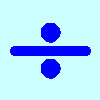### Division Rules

##### Age 7 to 11Challenge Level

This challenge encourages you to explore dividing a three-digit number by a single-digit number.### Abundant Numbers

##### Age 7 to 11Challenge Level

48 is called an abundant number because it is less than the sum of its factors (without itself). Can you find some more abundant numbers?### A Square of Numbers

##### Age 7 to 11Challenge Level

Can you put the numbers 1 to 8 into the circles so that the four calculations are correct?### Times Tables Shifts

##### Age 7 to 11Challenge Level

In this activity, the computer chooses a times table and shifts it. Can you work out the table and the shift each time?### Always, Sometimes or Never? Number

##### Age 7 to 11Challenge Level

Are these statements always true, sometimes true or never true?### Odd Squares

##### Age 7 to 11Challenge Level

Think of a number, square it and subtract your starting number. Is the number you're left with odd or even? How do the images help to explain this?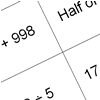### How Do You Do It?

##### Age 7 to 11Challenge Level

This group activity will encourage you to share calculation strategies and to think about which strategy might be the most efficient.##### Age 7 to 11Challenge Level

Can you match these calculation methods to their visual representations?### Flashing Lights

##### Age 7 to 11Challenge Level

Norrie sees two lights flash at the same time, then one of them flashes every 4th second, and the other flashes every 5th second. How many times do they flash together during a whole minute?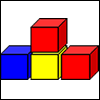### Up and Down Staircases

##### Age 7 to 11Challenge Level

One block is needed to make an up-and-down staircase, with one step up and one step down. How many blocks would be needed to build an up-and-down staircase with 5 steps up and 5 steps down?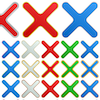### Compare the Calculations

##### Age 7 to 11Challenge Level

Can you put these four calculations into order of difficulty? How did you decide?### The Moons of Vuvv

##### Age 7 to 11Challenge Level

The planet of Vuvv has seven moons. Can you work out how long it is between each super-eclipse?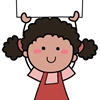### Carrying Cards

##### Age 7 to 11Challenge Level

These sixteen children are standing in four lines of four, one behind the other. They are each holding a card with a number on it. Can you work out the missing numbers?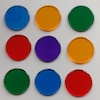### Dicey Array

##### Age 5 to 11Challenge Level

Watch the video of this game being played. Can you work out the rules? Which dice totals are good to get, and why?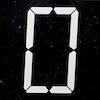### An Easy Way to Multiply by 10?

##### Age 7 to 11Challenge Level

Do you agree with Badger's statements? Is Badger's reasoning 'watertight'? Why or why not?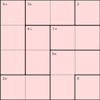### 4 by 4 Mathdokus

##### Age 7 to 14Challenge Level

Can you use the clues to complete these 4 by 4 Mathematical Sudokus?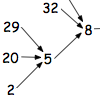##### Age 7 to 11Challenge Level

What happens when you add the digits of a number then multiply the result by 2 and you keep doing this? You could try for different numbers and different rules.### Factors and Multiples Game

##### Age 7 to 16Challenge Level

A game in which players take it in turns to choose a number. Can you block your opponent?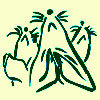### Music to My Ears

##### Age 7 to 11Challenge Level

Can you predict when you'll be clapping and when you'll be clicking if you start this rhythm? How about when a friend begins a new rhythm at the same time?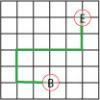### Journeys in Numberland

##### Age 7 to 11Challenge Level

Tom and Ben visited Numberland. Use the maps to work out the number of points each of their routes scores.### Multiplication Squares

##### Age 7 to 11Challenge Level

Can you work out the arrangement of the digits in the square so that the given products are correct? The numbers 1 - 9 may be used once and once only.### Multiplication Square Jigsaw

##### Age 7 to 11Challenge Level

Can you complete this jigsaw of the multiplication square?### What's in the Box?

##### Age 7 to 11Challenge Level

This big box multiplies anything that goes inside it by the same number. If you know the numbers that come out, what multiplication might be going on in the box?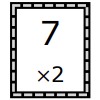### Ordering Cards

##### Age 5 to 11Challenge Level

This problem is designed to help children to learn, and to use, the two and three times tables.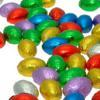### Let Us Divide!

##### Age 7 to 11Challenge Level

Look at different ways of dividing things. What do they mean? How might you show them in a picture, with things, with numbers and symbols?### Sweets in a Box

##### Age 7 to 11Challenge Level

How many different shaped boxes can you design for 36 sweets in one layer? Can you arrange the sweets so that no sweets of the same colour are next to each other in any direction?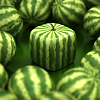### Shape Times Shape

##### Age 7 to 11Challenge Level

These eleven shapes each stand for a different number. Can you use the number sentences to work out what they are?### Multiply Multiples 1

##### Age 7 to 11Challenge Level

Can you complete this calculation by filling in the missing numbers? In how many different ways can you do it?### Round and Round the Circle

##### Age 7 to 11Challenge Level

What happens if you join every second point on this circle? How about every third point? Try with different steps and see if you can predict what will happen.### Remainders

##### Age 7 to 14Challenge Level

I'm thinking of a number. My number is both a multiple of 5 and a multiple of 6. What could my number be?### Picture a Pyramid ...

##### Age 7 to 11Challenge Level

Imagine a pyramid which is built in square layers of small cubes. If we number the cubes from the top, starting with 1, can you picture which cubes are directly below this first cube?### Make 100

##### Age 7 to 11Challenge Level

Find at least one way to put in some operation signs (+ - x Ã·) to make these digits come to 100.### Table Patterns Go Wild!

##### Age 7 to 11Challenge Level

Nearly all of us have made table patterns on hundred squares, that is 10 by 10 grids. This problem looks at the patterns on differently sized square grids.### Counting Cogs

##### Age 7 to 14Challenge Level

Which pairs of cogs let the coloured tooth touch every tooth on the other cog? Which pairs do not let this happen? Why?### Mystery Matrix

##### Age 7 to 11Challenge Level

Can you fill in this table square? The numbers 2 -12 were used to generate it with just one number used twice.### Light the Lights Again

##### Age 7 to 11Challenge Level

Each light in this interactivity turns on according to a rule. What happens when you enter different numbers? Can you find the smallest number that lights up all four lights?### Multiples Grid

##### Age 7 to 11Challenge Level

What do the numbers shaded in blue on this hundred square have in common? What do you notice about the pink numbers? How about the shaded numbers in the other squares?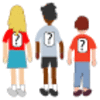### One Wasn't Square

##### Age 7 to 11Challenge Level

Mrs Morgan, the class's teacher, pinned numbers onto the backs of three children. Use the information to find out what the three numbers were.### All the Digits

##### Age 7 to 11Challenge Level

This multiplication uses each of the digits 0 - 9 once and once only. Using the information given, can you replace the stars in the calculation with figures?### Factor Track

##### Age 7 to 14Challenge Level

Factor track is not a race but a game of skill. The idea is to go round the track in as few moves as possible, keeping to the rules.### Factor Lines

##### Age 7 to 14Challenge Level

Arrange the four number cards on the grid, according to the rules, to make a diagonal, vertical or horizontal line.### Two Primes Make One Square

##### Age 7 to 11Challenge Level

Can you make square numbers by adding two prime numbers together?### Factor-multiple Chains

##### Age 7 to 11Challenge Level

Can you see how these factor-multiple chains work? Find the chain which contains the smallest possible numbers. How about the largest possible numbers?### Pebbles

##### Age 7 to 11Challenge Level

Place four pebbles on the sand in the form of a square. Keep adding as few pebbles as necessary to double the area. How many extra pebbles are added each time?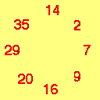### Cycling Squares

##### Age 7 to 11Challenge Level

Can you make a cycle of pairs that add to make a square number using all the numbers in the box below, once and once only?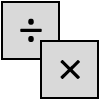### Four Go

##### Age 7 to 11Challenge Level

This challenge is a game for two players. Choose two of the numbers to multiply or divide, then mark your answer on the number line. Can you get four in a row?### Four Goodness Sake

##### Age 7 to 11Challenge Level

Use 4 four times with simple operations so that you get the answer 12. Can you make 15, 16 and 17 too?### Curious Number

##### Age 7 to 11Challenge Level

Can you order the digits from 1-3 to make a number which is divisible by 3 so when the last digit is removed it becomes a 2-figure number divisible by 2, and so on?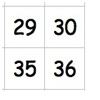### So It's Times!

##### Age 7 to 14Challenge Level

How will you decide which way of flipping over and/or turning the grid will give you the highest total?### Cubes Within Cubes

##### Age 7 to 14Challenge Level

We start with one yellow cube and build around it to make a 3x3x3 cube with red cubes. Then we build around that red cube with blue cubes and so on. How many cubes of each colour have we used?